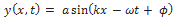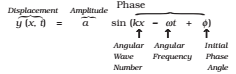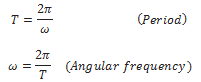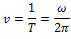## Progressive Wave:

A wave that travels from one point of the medium to another is called a progressive wave. A progressive wave may be transverse or longitudinal.

## Plane progressive harmonic wave:

If during the propagation of a wave through a medium, the particles of the medium vibrate simply harmonically about their mean positions, then the wave is said to be a plane progressive harmonic wave.

## Displacement relation for a progressive harmonic wave:

The displacement in a sinusoidal wave propagating in the positive x-direction is given bywhere a is the amplitude of the wave, k is the angular wave number, ω is the angular frequency, (kx – ωt + φ) is the phase, and φ is the phase constant or phase angle.

The sine function and the time-dependent phase of a wave correspond to the oscillation of a string element, and the amplitude of the wave determines the extremes of the element’s displacement. The constant ϕ is called the initial phase angle.

## Wavelength

Wavelength λ of a progressive wave is the distance between two consecutive points of the same phase at a given time. In a stationary wave, it is twice the distance between two consecutive nodes or antinodes. k is called the propagation constant or the angular wave number.